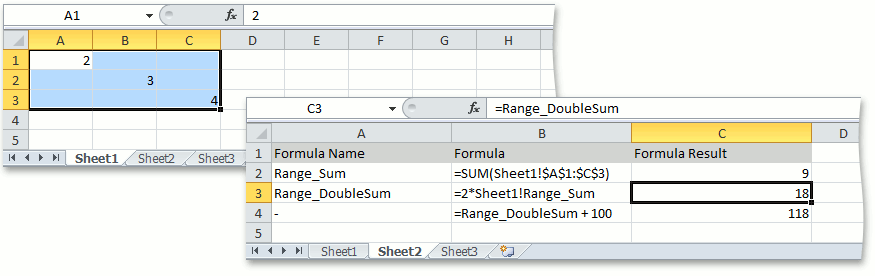21.1
20.2
20.1
19.2
19.1
18.2
18.1
17.2
.NET Framework 4.5.2+
.NET Framework 4.5.2+
.NET Standard 2.0+
A newer version of this page is available. Switch to the current version.

# How to: Create Named Formulas

• 2 minutes to read

This example demonstrates how to define names for formulas. To do this, call the DefinedNameCollection.Add method with a name to be associated with a formula and the formula string passed as parameters. Use the Worksheet.DefinedNames or Workbook.DefinedNames property to access and modify the collection of defined names of a particular worksheet or entire workbook, depending on which scope you want to specify for a name.

##### NOTE

A complete sample project is available at https://github.com/DevExpress-Examples/spreadsheet-document-server-api-e4339

``````Worksheet worksheet1 = workbook.Worksheets["Sheet1"];
Worksheet worksheet2 = workbook.Worksheets["Sheet2"];

// Create a name for a formula that sums up the values of all cells included in the "A1:C3" range of the "Sheet1" worksheet.
// The scope of this name will be limited by the "Sheet1" worksheet.
worksheet1.DefinedNames.Add("Range_Sum", "=SUM(Sheet1!\$A\$1:\$C\$3)");

// Create a name for a formula that doubles the value resulting from the "Range_Sum" named formula and
// make this name available within the entire workbook.
workbook.DefinedNames.Add("Range_DoubleSum", "=2*Sheet1!Range_Sum");

// Create formulas that use other formulas with the specified names.
worksheet2.Cells["C2"].Formula = "=Sheet1!Range_Sum";
worksheet2.Cells["C3"].Formula = "=Range_DoubleSum";
worksheet2.Cells["C4"].Formula = "=Range_DoubleSum + 100";
``````

The image below shows how to use named formulas in worksheet cells (the workbook is opened in Microsoft® Excel®).See Also